# AP Chemistry : History and Experiments with Electrons

## Example Questions

### Example Question #1 : Electrons And Quantum Theory

What is the de Broglie wavelength (in meters) of a person walking? Assume a person walks at 4 mph and has a mass of 80 kg.

4.6e-36

5.8e-34

2.6e-36

3.6e-34

6.8e-38

4.6e-36

Explanation:

de Broglie suggested that all matter has both wave-like and particle-like properties. His
equation states that λ= h/mv , where λ is the wavelength, m is mass, h is Planck’s constant,
and v is velocity. 4 mph is 1.8 m s−1 and Planck’s constant is 6.626e-34 kg m2 s−1 , giving
us a wavelength (λ) 4.6e-36 m ! A very small wavelength, indeed.

### Example Question #101 : Ap Chemistry

Ernest Rutherford's famous gold foil experiment demonstrated which of the following principles?

Neutral atoms contain an equal number of protons and electrons

Atoms contain a small dense nucleus with an equal number of protons and neutrons

Atoms have an even distribution of positive charge and scattered negative charge

Each atom contains a small, dense, positively charged nucleus

Each atom contains a small, dense, positively charged nucleus

Explanation:

Rutherfords experiment focused a beam of alpha particles on a piece of gold foil. The result showed that most of the alpha particles passed through the foil, while a small fraction of particles were significantly deflected. This suggested the presence of a small, dense, positively charged nucleus. Most of the alpha particles passed through the electron clouds of the gold atoms, without impacting the nuclei, while those that did impact the nuclei were deflected by the positive charge of the nucleus.

Rutherford's experiment does not give us any concrete information about neutrons, nor does it allow us to assume that the number of protons and neutrons in the nucleus are equal.

### Example Question #102 : Ap Chemistry

Which scientist conducted an experiment studying the structure of the atom by firing alpha particles at a thin gold foil?

Niels Bohr

J.J. Thomson

Max Planck

Ernest Rutherford

Ernest Rutherford

Explanation:

Ernest Rutherford made great advances in current understanding of atomic structure through his gold foil experiment. When firing positively-charged alpha particles at a thin film of gold atoms, most particles were found to pass straight through the film with little to no deflection, indicating that atoms were mostly composed of empty space. A few particles were deflected at large angles, indicating direct collisions with the positively charged nuclei of the atoms.

### Example Question #103 : Ap Chemistry

What did the Rutherford gold foil experiment show?

Protons, neutrons, and electrons are evenly dispersed in the space occupied by an atom

Protons have a charge of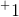, while electrons have a charge of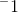and neutrons are uncharged

Protons and neutrons are similar in mass, while electrons have a significantly smaller mass

The positively-charged atomic nucleus is concentrated to a tiny fraction of the total atomic volume, most of which is occupied by the electron cloud

The positively-charged atomic nucleus is concentrated to a tiny fraction of the total atomic volume, most of which is occupied by the electron cloud

Explanation:

In the Rutherford experiment, a beam of alpha particles was shot through a gold foil, with most of the particles flying straight through and a few scattering at angles greater than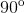. These results suggest that most of the volume of foil was "empty" space, with small concentrations of positive charge, which we now know is the nucleus.

### Example Question #104 : Ap Chemistry

Which of the following statements regarding the discovery of the electron are true?

From the oil drop experiment, Dalton calculated the mass of the electron to be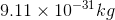J.J. Thomson was able to deduce the mass of the electron following his experiments using oil drops

J.J. Thomson's cathode ray tube experiment proved the existence of a small negatively charged particle, which he named the electron

Robert Millikan's cathode ray tube experiment proved the existence of a small negatively charged particle, which he named the electron

J.J. Thomson's cathode ray tube experiment proved the existence of a small negatively charged particle, which he named the electron

Explanation:

Recall that J.J. Thomson conducted the cathode ray tube experiment that proved the existence of a small negatively charged particle. Thomson was also able to determine the charge-to-mass ratio of an electron. Robert Millikan is the scientist who conducted the oil drop experiment, from which he was able to find the charge of the electron and deduce its mass using the electron's charge-to-mass ratio. Dalton was not involved in the discovery of the electron.

### Example Question #105 : Ap Chemistry

From Robert Millikan's oil drop experiment, the charge of the electron was observed to be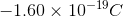. Which of the following charges could not have been observed from the oil drop experiment?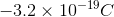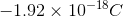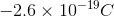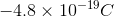Explanation:

All the oil drops must have a charge that is a multiple of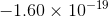. If an oil drop is observed to have a charge of, that means the oil drop has three electrons. If an oil drop is observed to have a charge of, that means the oil drop has twelve electrons. If an oil drop is observed to have a charge of, that means the oil drop has two electrons.

### Example Question #106 : Ap Chemistry

From Robert Millikan's oil drop experiment, the charge of the electron was observed to be. Suppose that one of the oil drops has a charge of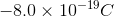. How many electrons does this oil drop contain?Explanation:

To find the number of electrons this oil drop contains, divide the observed charge by the charge of a single electron.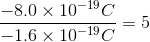This oil drop containselectrons.

### Example Question #107 : Ap Chemistry

Which of the following statements concerning the discovery of the electron are false?

Using the charge-to-mass ratio, Robert Millikan was able to deduce the mass of the electron

Robert Millikan was able to find the charge-to-mass ratio of the electron after conducting experiments using cathode ray tubes

J.J. Thomson was able to find the charge-to-mass ratio of the electron after conducting experiments using cathode ray tubes

J.J. Thomson proved the existence of the electron when he showed that the cathode rays were repelled by the negative pole of an electric field

Robert Millikan was able to find the charge-to-mass ratio of the electron after conducting experiments using cathode ray tubes

Explanation:

Recall that J.J. Thomson conducted the cathode ray tube experiment that proved the existence of a small negatively charged particle. Thomson was also able to determine the charge-to-mass ratio of an electron. Robert Millikan is the scientist who conducted the oil drop experiment, from which he was able to find the charge of the electron and deduce its mass using the electron's charge-to-mass ratio.

### Example Question #1 : Elements And Atoms

Which of the following is a result of J.J. Thomson's cathode ray tube experiment?

The plum pudding model of atoms was postulated

The mass of an electron was calculated

The charge of an electron was calculated

The neutron was discovered